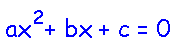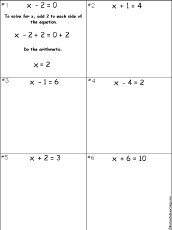EnchantedLearning.com is a user-supported site.
As a bonus, site members have access to a banner-ad-free version of the site, with print-friendly pages.

 You might also like: Algebra: Solve the quadratic equations Worksheet #4 Printout Algebra: Solve the quadratic equations Worksheet #6 Printout Algebra: Solve the quadratic equations Worksheet #1 Printout Algebra: Multiplying Polynomials Worksheet #2 Printout Algebra Glossary - Terms Used in Algebra Today's featured page: Reducing Fractions to Lowest TermsMore Math Activities EnchantedLearning.comAlgebraAlgebra is the study of generalized arithmetic. Algebra lets you solve puzzles and problems that would be very difficult to solve otherwise. In algebra, unknown numbers can be represented by letters (called variables) in order to solve equations. For example, 4 + x = 10 is true when x=6.

Variables together with mathematical symbols and operations can replace verbal statements. For example, the statement, "The area of a rectangle is the product of the legth and the width" can be summarized by the algebraic statement, "A=l x w."

This is a practical example of algebra being useful. If you want to go to a gym occasionally, but need to decide if you should pay \$5 per visit or get a yearly pass for \$100. Which option is best for you? This depends on how often you plan to go to the gym, but in order to make your decision, you need to figure out at which number visits these options are equal. If you go more often than this many times, you should get a membership. You can solve this problem using algebra. Let v equal the number of visits.
 5v = 100 v = 20
So, if you plan on going more than 20 times this year, joining the gym will save you money. If you plan on going fewer than 20 times this year, don't join, simply pay the \$5 per visit fee to save money.

Algebra (originally called al-jabr, meaning "restoration") was invented in the Middle East by Abu Ja'far Muhammad ibn Musa Al-Khwarizmi (born in Baghdad about AD 825) during the Middle Ages.

Algebra Topics:

1. Introduction to Algebra:
• Symbols Used in Arithmetic for Operations (addition +, subtraction -, multiplication x, (), or no sign, division ÷, /)
• Order of Operations
• Commutative Property (plus using it t o simply addition anad multiplication)
• Associative Property
• Multiplicative Inverse
• Distributive Property
• Variables - representing numbers by letters
• Coefficients
• Expressions (plus replacing verbal statements with expressions for addition, subtraction, multiplication, and division)
• Adding and Subtracting Variables (plus stating products without using multiplication sign)
• Multiplying and Dividing Variables (plus not dividing by zero)
• Parentheses
• Factors
• Base to an Exponent Equals a Power
• Like and Unlike Terms
• Absolute Value
• Terms Used in Algebra
2. Simple Equations and How to Solve Them
• ccc

Worksheets:
Introduction to Albebra - Worksheets to PrintSolve Linear Equations WorksheetSolve 10 simple linear equations by adding or subtracting a constant from each side of the equation. These problems all have a positive number as an answer. Worksheet #1 or go to the answers. Worksheet #2 or go to the answers. Worksheet #3 or go to the answers. Worksheet #4 or go to the answers. Worksheet #5 or go to the answers.Solve Linear Equations Worksheet: Negative Number AnswerSolve 10 simple linear equations by adding or subtracting a constant from each side of the equation. These problems all have a negative number as an answer. Worksheet #1 or go to the answers. Worksheet #2 or go to the answers. Worksheet #3 or go to the answers. Worksheet #4 or go to the answers. Worksheet #5 or go to the answers.Multiply Polynomials Worksheet #1Multiply 10 polynomials and put the answers in simplest form. Or go to the answers.Multiply Polynomials Worksheet #2Multiply 10 polynomials and put the answers in simplest form. Or go to the answers. Quadratic Equation: ax2 - c = 0Printable Worksheets
 +, - EnchantedLearning.comMath x, ÷
 A B C D E F G H I J K L M N O P Q R S T U V W X Y Z
 Number Line Fractions Decimals Measurement Rounding Graphs

Enchanted Learning®
Over 35,000 Web Pages
Sample Pages for Prospective Subscribers, or click below

 Overview of Site What's New Enchanted Learning Home Monthly Activity Calendar Books to Print Site Index K-3 Crafts K-3 Themes Little ExplorersPicture dictionary PreK/K Activities Rebus Rhymes Stories Writing Cloze Activities Essay Topics Newspaper Writing Activities Parts of Speech Fiction The Test of Time iPhone app TapQuiz Maps - free iPhone Geography Game Biology Animal Printouts Biology Label Printouts Biomes Birds Butterflies Dinosaurs Food Chain Human Anatomy Mammals Plants Rainforests Sharks Whales Physical Sciences: K-12 Astronomy The Earth Geology Hurricanes Landforms Oceans Tsunami Volcano Languages Dutch French German Italian Japanese (Romaji) Portuguese Spanish Swedish Geography/History Explorers Flags Geography Inventors US History Other Topics Art and Artists Calendars College Finder Crafts Graphic Organizers Label Me! Printouts Math Music Word Wheels

## Enchanted Learning Search

 Search the Enchanted Learning website for: